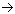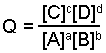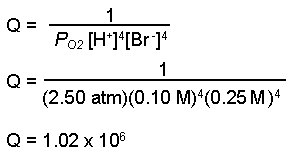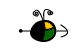Electrochemical Cell Potentials

The cell potential (voltage) for an electrochemical cell can be predicted from half-reactions and its operating conditions (chemical nature of materials, temperature, gas partial pressures, and concentrations).

Determining Standard State Cell Potentials

A cell's standard state potential is the potential of the cell under standard state conditions, which is approximated with concentrations of 1 mole per liter (1 M) and pressures of 1 atmosphere at 25oC.

To calculate the standard cell potential for a reaction

• Write the oxidation and reduction half-reactions for the cell.
• Look up the reduction potential, Eoreduction,  for the reduction half-reaction in a table of reduction potentials
• Look up the reduction potential for the reverse of the oxidation half-reaction and reverse the sign to obtain the oxidation potential.  For the oxidation half-reaction, Eooxidation = - Eoreduction.
• Add the potentials of the half-cells to get the overall standard cell potential.
Eocell = Eoreduction + Eooxidation

Example:  Find the standard cell potential for an electrochemical cell with the following cell reaction.

Zn(s) + Cu2+(aq)Zn2+(aq) + Cu(s)

• Write the half-reactions for each process.
• Zn(s)Zn2+(aq) + 2 e-

Cu2+(aq) + 2 e-Cu(s)

• Look up the standard potentials for the redcution half-reaction.
• Eoreduction of Cu2+ = + 0.339 V

• Look up the standard reduction potential for the reverse of the oxidation reaction and change the sign.
• Eoreduction of Zn2+ = - 0.762 V

Eooxidation of Zn = - ( - 0.762 V) = + 0.762 V

• Add the cell potentials together to get the overall standard cell potential.

 oxidation: Zn(s)Zn2+(aq) + 2 e- Eoox. =  - Eored. = - (- 0.762 V) = + 0.762 V reduction: Cu2+(aq) + 2 e-Cu(s) Eored. = + 0.339 V overall: Zn(s) + Cu2+(aq)Zn2+(aq) + Cu(s) Eocell = + 1.101 V

Top

Determining Non-Standard State Cell Potentials

To determine the cell potential when the conditions are other than standard state (concentrations not 1 molar and/or pressures not 1 atmosphere):

• Determine the standard state cell potential.
• Determine the new cell potential resulting from the changed conditions.
• Determine Q, the reaction quotient.
• Deternine n, the number of electrons transferred in the reaction "n".
• Determine Ecell, the cell potential at the non-standard state conditions using the Nernst equation.
Ecell = Eocell - (RT/nF) ln Q

Ecell = cell potential at non-standard state conditions
Eocell = standard state cell potential
R = constant (8.31 J/mole K)
T = absolute temperature (Kelvin scale)
F = Faraday's constant (96,485 C/mole e-)
n = number of moles of electrons transferred in the balanced equation for the reaction occurring
in the cell
Q = reaction quotient for the reaction.  aA + bBcC + dD,If the temperature of the cell remains at 25oC, the equation simplifies to:

Ecell = Eocell - (0.0257/n) ln Q

or in terms of log10

Ecell = Eocell - (0.0592/n) log Q

Example:  Predict the cell potential for the following reaction when the pressure of the oxygen gas is 2.50 atm, the hydrogen ion concentration is 0.10 M, and the bromide ion concentration is 0.25 M.

O2(g) + 4 H+(aq) + 4 Br-(aq)2 H2O(l) + 2 Br2(l)

• Calculate the standard cell potential for the reaction, Eocell, using the tabled values:
•  oxidation: 4 Br-(aq)2 Br2(l) + 4 e- Eoox. = - Eored. = - (+ 1.077 V) = - 1.077 V reduction: O2(g) + 4 H+(aq) + 4 e-2 H2O(l) Eored. = + 1.229 V overall: O2(g) + 4 H+(aq) + 4 Br-(aq)2 H2O(l) + 2 Br2(l) Eocell = + 0.152 V

• Determine the new cell potential resulting from the changed conditions.
• Calculate the value for the reaction quotient, Q.  (Note: We calculate Q using molar concentrations for solutions and pressures for gases.  Water and bromine are both liquids, therefore they are not included in the calculation of Q.)
•• Calculate the number of moles of electrons transferred in the balanced equation, n.
• n = 4 moles of electrons

• Substitute values into the Nernst equation and solve for the non-standard cell potential, Ecell.

Ecell = + 0.152 V - (0.0257/4) ln(1.02 x 106)
Ecell = 0.063 V